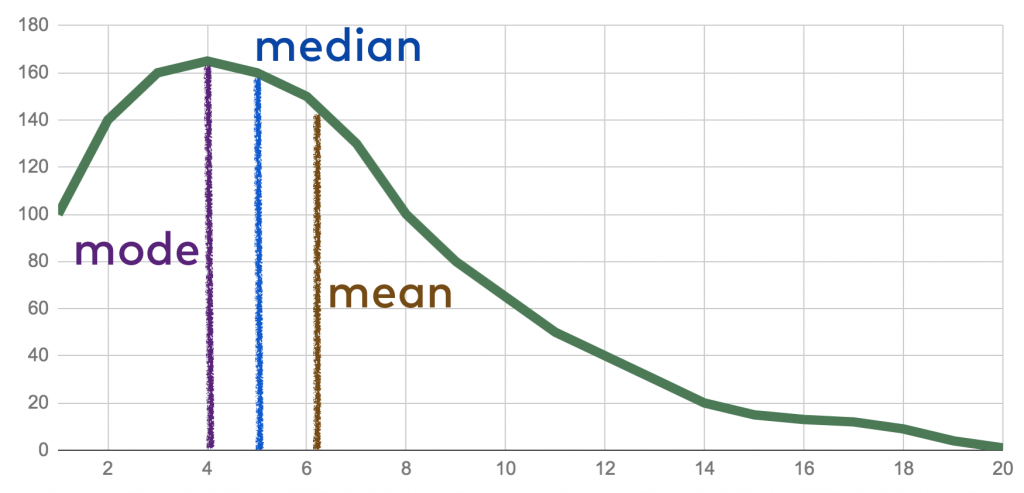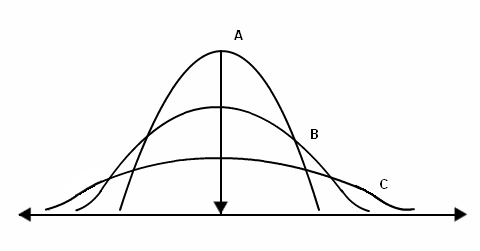Maths Topic Statistics for SSC-CGL Exam - Study24x7New to Study24X7 ?

# Maths Topic Statistics for SSC-CGL Exam

Updated on 02 July 2021SSC 2021 Preparation Strategie
Updated on 02 July 2021The aspirants who are preparing for SSC CGL must understand what SSC CGL is.

The SSC CGL age limit and syllabus for SSC CGL has been already mentioned on their website https://ssc.nic.in/

Collection Classification and Presentation of Statistical Data for SSC-CGL

In statistics, the raw data is collected and classified on the basis of different categorizations to simplify the further process.

Collection of Statistical Data: Data can be collected in three ways:

## Questionnaire method

1. Interview method
2. Registration method

Classification of Statistical Data:

Classification of statistical data includes the process of aligning data into a particular order on the basis of some common characteristic from the available raw data.

## In statistics, data can be classified in the following ways:

1. Qualitative Classification

2. Quantitative Classification

3. Chronological Classification

4. Geographical Classification

5. Conditional Classification

Representation of data:

Textural Method: This method is used on data for synthesis and generalization purposes.

Tabular Method: It represents the data in row and column-wise and provides a way to compare it easily.

Semi tabular Method: It includes both text and tables in its representation.

Graphical Representation: It represents the data in the form of different charts or graphs like a pie chart, bar graph, etc.

Measures of Central Tendency for SSC CGL

It is a single representation of a mass set of data.

There are three main measures of central tendency.

These measures are mean, median, mode.

Mean: It gives a single value for a mass set of data by adding all observations present in the dataset and divide it by the total number of values in the dataset. Here scope for estimation is not present.

Mean - addition of all observations/ total number of observations

Median - It gives the middle value of an arranged dataset where an arrangement of the values is in ascending order.

When a dataset has an odd number of observations:

Median = Middle value of dataset

When the dataset has an even number of observations:

Median= Mean of two middle values

It divides the distribution of the dataset in half.

Mode: It is the value defined on the basis of the occurrence of any value in the dataset. It gives the value of the most observed data element in the dataset.

It can be determined for both quantitative and categorical types of data.

It cannot represent the center of distribution for a dataset.Measures of Dispersion for CGL

It represents the scattering of data. It shows the deviation of data from the central distribution.

It is used to compare different data sets and series.

Different measures of dispersion are as follows:

Range

Quartile Deviation

Interquartile range

Mean Deviation

Standard Deviation

Lorenz Curve

There are two categories of measure of dispersion.

These are as follows:

The absolute measure of dispersion: It is measured in terms of actual values of datasets.

For example,

Range= Dmax- Dmin

Where D is the distance between two values.

A relative measure of dispersion: It is used for comparing the two or more data set of different units. It is represented in the form of the ratio of averages.Hope you find our article for your SSC-CGL Preparation!

Write a comment...Trending ArticlesBy CBSE CLASS 8By CBSE CLASS 10By Examination GuideBy K-12-Commerce
Related Posts